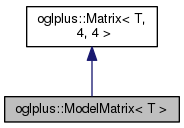OGLplus (0.52.0) a C++ wrapper for OpenGLoglplus::ModelMatrix< T > Class Template Reference

Class implementing model transformation matrix named constructors. More...

`#include </home/chochlik/devel/oglplus/include/oglplus/math/matrix.hpp>`

Inheritance diagram for oglplus::ModelMatrix< T >:[legend]
Collaboration diagram for oglplus::ModelMatrix< T >:[legend]

## Public Member Functions

ModelMatrix (void)
Constructs an identity matrix.

const TData (void) const
Returns a pointer to the matrix elements in row major order.

std::size_t Size (void) const
Returns the number of elements of the matrix.

T At (std::size_t i, std::size_t j) const
Returns the value of the element at position i, j. More...

void Set (std::size_t i, std::size_t j, T v)
Sets the value of the element at position i, j. More...

Vector< T, ColsRow (std::size_t i) const
Returns the i-th row of this matrix. More...

Vector< T, RowsCol (std::size_t j) const
Return the j-th column of this matrix. More...

Matrix< T, R, C > Submatrix (void) const
Submatrix extraction.

## Static Public Member Functions

static ModelMatrix Translation (T dx, T dy, T dz)
Constructs a translation matrix.

static ModelMatrix TranslationX (T dx)
Constructs a translation matrix.

static ModelMatrix TranslationY (T dy)
Constructs a translation matrix.

static ModelMatrix TranslationZ (T dz)
Constructs a translation matrix.

static ModelMatrix Translation (const Vector< T, 3 > &dp)
Constructs a translation matrix.

static ModelMatrix Scale (T sx, T sy, T sz)
Constructs a scale matrix.

static ModelMatrix Reflection (bool rx, bool ry, bool rz)
Constructs a reflection matrix.

static ModelMatrix RotationX (Angle< T > angle)
Constructs a X-axis rotation matrix.

static ModelMatrix RotationY (Angle< T > angle)
Constructs a Y-axis rotation matrix.

static ModelMatrix RotationZ (Angle< T > angle)
Constructs a Z-axis rotation matrix.

static ModelMatrix RotationA (const Vector< T, 3 > &axis, Angle< T > angle)
Constructs a rotation matrix from a vector and angle.

static ModelMatrix RotationQ (const Quaternion< T > &quat)
Constructs a rotation matrix from a quaternion.

## Detailed Description

### template<typename T> class oglplus::ModelMatrix< T >

Class implementing model transformation matrix named constructors.

The static member functions of this class can be used to construct various model transformation matrices.

## Member Function Documentation

 T oglplus::Matrix< T, Rows, Cols >::At ( std::size_t i, std::size_t j ) const
inherited

Returns the value of the element at position i, j.

Precondition
(i < Rows) && (j < Cols)
 Vector oglplus::Matrix< T, Rows, Cols >::Col ( std::size_t j ) const
inherited

Return the j-th column of this matrix.

Precondition
(j < Cols)
 Vector oglplus::Matrix< T, Rows, Cols >::Row ( std::size_t i ) const
inherited

Returns the i-th row of this matrix.

Precondition
(i < Rows)
 void oglplus::Matrix< T, Rows, Cols >::Set ( std::size_t i, std::size_t j, T v )
inherited

Sets the value of the element at position i, j.

Precondition
(i < Rows) && (j < Cols)
Examples:
oglplus/021_translucent_arrow.cpp, oglplus/022_blender_mesh.cpp, oglplus/028_monkeycraft.cpp, oglplus/031_fog.cpp, and oglplus/032_object_tracking.cpp.

The documentation for this class was generated from the following file:
• /home/chochlik/devel/oglplus/include/oglplus/math/matrix.hpp